Next: Linear Stark Effect Up: Time-Independent Perturbation Theory Previous: Quadratic Stark Effect

# Degenerate Perturbation Theory

Let us now consider systems in which the eigenstates of the unperturbed Hamiltonian,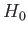, possess degenerate energy levels. It is always possible to represent degenerate energy eigenstates as the simultaneous eigenstates of the Hamiltonian and some other Hermitian operator (or group of operators). Let us denote this operator (or group of operators)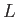. We can write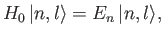(7.66)

and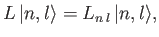(7.67)

where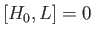. Here, the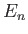and the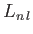are real numbers that depend on the quantum numbers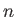, andand, respectively. It is always possible to find a sufficient number of operators that commute with the Hamiltonian in order to ensure that theare all different. In other words, we can choosesuch that the quantum numbersanduniquely specify each eigenstate. Suppose that for each value ofthere are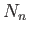different values of. In other words, theth energy eigenstate is-fold degenerate.

In general,does not commute with the perturbing Hamiltonian,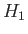. In this situation, we expect the perturbation to couple degenerate states with the same value of, but different values of. Let us naively attempt to use the standard perturbation theory of Section 7.3 to evaluate the modified energy eigenstates and energy levels. A direct generalization of Equations (7.32) and (7.33) yields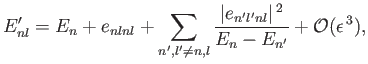(7.68)

and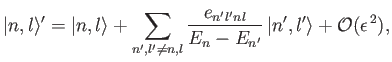(7.69)

where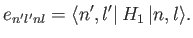(7.70)

It is fairly obvious that the summations in Equations (7.69) and (7.70) are not well behaved if theth energy level is degenerate. The problem terms are those that involve coupling to unperturbed eigenstates labeled by the same value of, but different values of: that is, those states whose unperturbed energies are. These terms give rise to singular factors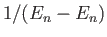in the summations. Note, however, that this problem would not exist if the matrix elements,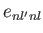, of the perturbing Hamiltonian between distinct, degenerate, unperturbed energy eigenstates corresponding to the eigenvaluewere zero. In other words, if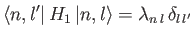(7.71)

then all of the singular terms in Equations (7.69) and (7.70) would vanish.

In general, Equation (7.72) is not satisfied. Fortunately, we can always redefine the unperturbed energy eigenstates belonging to the eigenvaluein such a manner that Equation (7.72) is satisfied. Let us definenew states that are linear combinations of theoriginal degenerate eigenstates corresponding to the eigenvalue: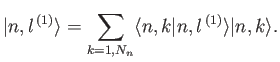(7.72)

Note that these new states are also degenerate energy eigenstates of the unperturbed Hamiltonian corresponding to the eigenvalue. The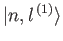are chosen in such a manner that they are eigenstates of the perturbing Hamiltonian,. Thus,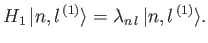(7.73)

Theare also chosen so that they are orthonormal. It follows that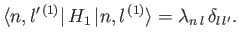(7.74)

Thus, if we use the new eigenstates, instead of the old ones, then we can employ Equations (7.69) and (7.70) directly, because all of the singular terms vanish. The only remaining difficulty is to determine the new eigenstates in terms of the original ones.

Now,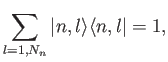(7.75)

where 1 denotes the identity operator in the sub-space of all unperturbed energy eigenkets corresponding to the eigenvalue. Using this completeness relation, the operator eigenvalue equation (7.74) can be transformed into a straightforward matrix eigenvalue equation: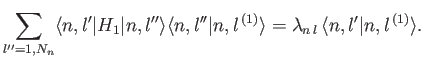(7.76)

This can be written more transparently as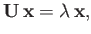(7.77)

where the elements of the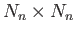Hermitian matrix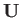are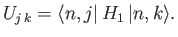(7.78)

Provided that the determinant ofis non-zero, Equation (7.78) can always be solved to giveeigenvalues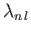(forto), withcorresponding eigenvectors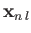. The eigenvectors specify the weights of the new eigenstates in terms of the original eigenstates: that is,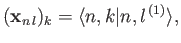(7.79)

for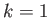to. In our new scheme, Equations (7.69) and (7.70) yield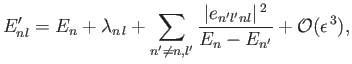(7.80)

and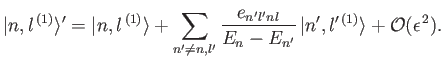(7.81)

There are no singular terms in these expressions, because the summations are over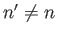. In other words, they specifically exclude the problematic, degenerate, unperturbed energy eigenstates corresponding to the eigenvalue. Note that the first-order energy-shifts are equivalent to the eigenvalues of the matrix equation (7.78).

Incidentally, it is clear, from the previous analysis, that if the perturbing Hamiltonian,, commutes withand the operator (or group of operators)then there is no danger of singular terms appearing in the perturbation expansion to second order, because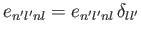. Another way of saying this is that there are no singular terms if the simultaneous eigenstates ofandare also eigenstates of.Next: Linear Stark Effect Up: Time-Independent Perturbation Theory Previous: Quadratic Stark Effect
Richard Fitzpatrick 2016-01-22